﻿ Bending channel with a porous media region - XSim

# Bending channel with a porous media region

Update: December 17, 2018
OpenFOAM 4.x

$FOAM_TUTORIALS/compressible/rhoPimpleFoam/ras/angledDuct ## Summary We calculate a flow with a porous material that models a filter in the middle of the flow path. The fluid flows in from the region "inlet" (end face of blue part) at a mass flow rate of 0.1 kg/s, passes through the filter (red part), and flows out from the region "outlet" (end face of green part).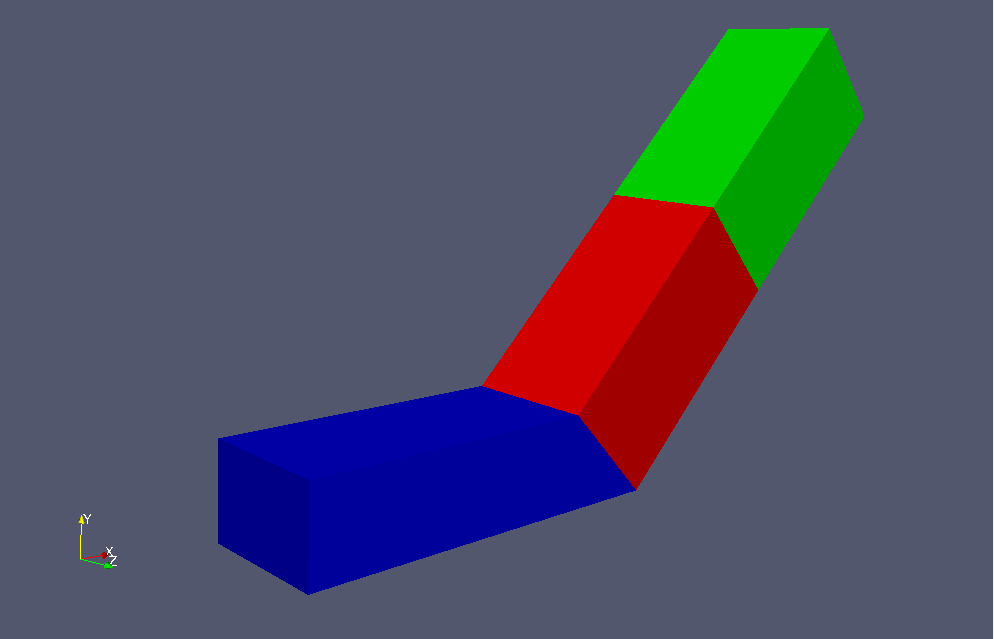Model geometry Porous media depends on the Darcy-Forchheimer law, and for a flow velocity ui (i=x, y, z) in direction i, a generation term Si (pressure drop) in the opposite direction of flow is added to the Navier-Stokes equations. Here, μ is the viscosity coefficient and ρ is the density. The parameters that determine the properties of the porous media, Dij, F, the direction of the properties, and the region in which the porous media, are specified in the file constant/fvOptions as follows. porosity1 { type explicitPorositySource; active yes; explicitPorositySourceCoeffs { selectionMode cellZone; cellZone porosity; type DarcyForchheimer; DarcyForchheimerCoeffs { d (7e5 -1000 -1000); f (0 0 0); coordinateSystem { type cartesian; origin (0 0 0); coordinateRotation { type axesRotation; e1 (0.70710678 0.70710678 0); e3 (0 0 1); } } } } }  The standard k-ε model is used as the turbulence model, and calculations are performed for 10 seconds. The meshes are as follows, and the number of mesh is 22000.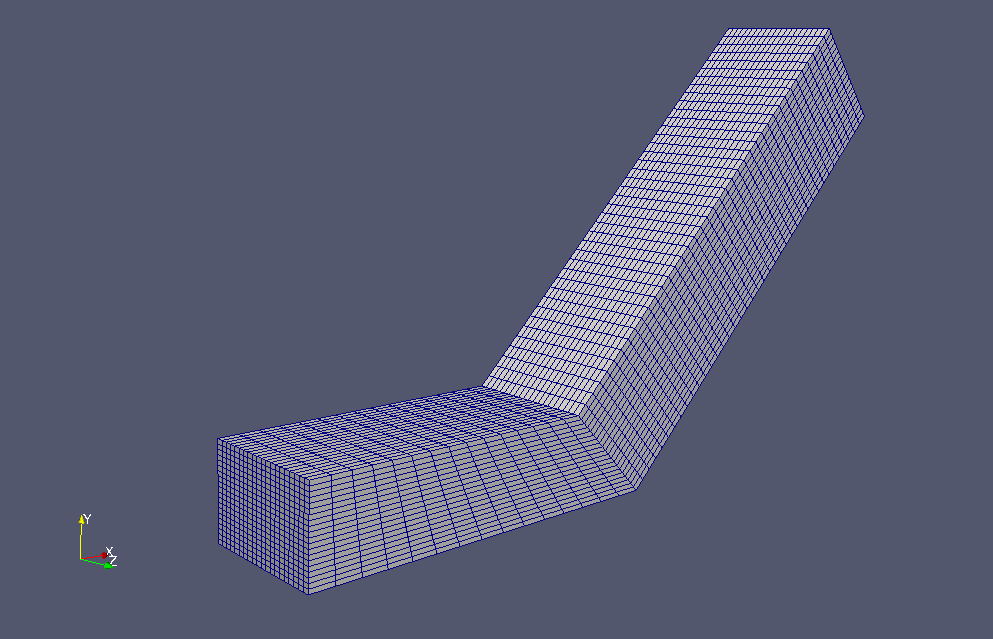Meshes If you want to visualize turbulent energy and turbulent dissipation rate, check "k" and "epsilon" in the "Properties" tab in ParaView.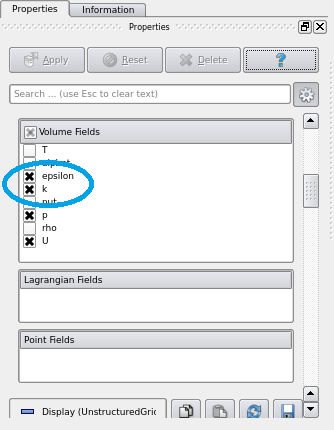Check "k" and "epsilon" in "Properties" tab The calculation result is as follows.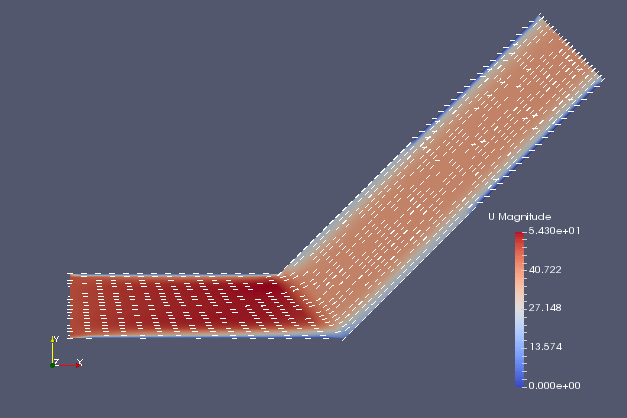Flow velocity (U)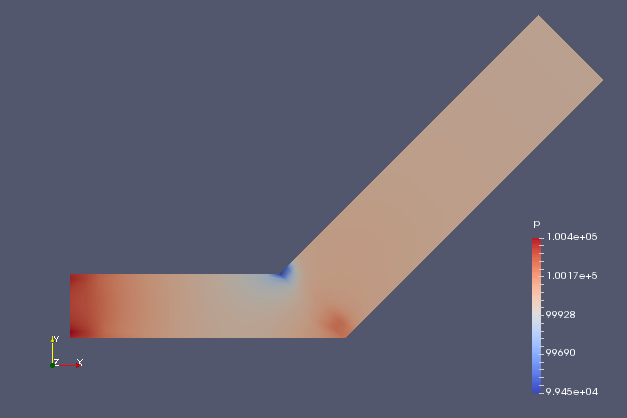Pressure (p)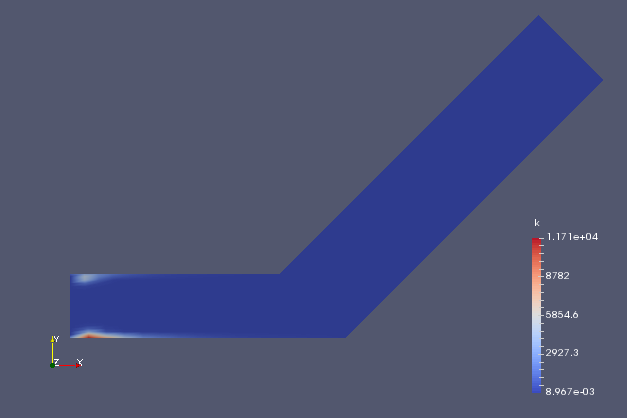Turbulent energy (k)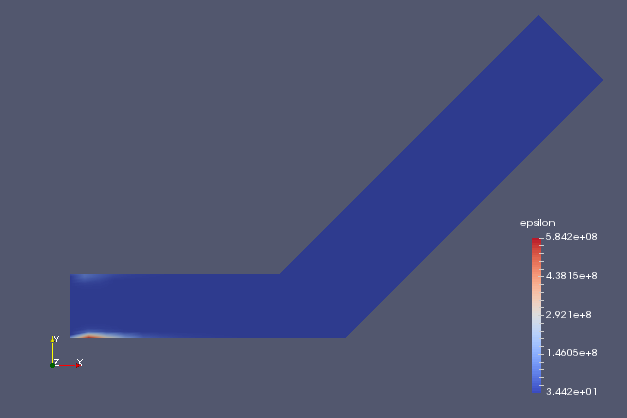Turbulent dissipation rate (epsilon) ## Commands cp -r$FOAM_TUTORIALS/compressible/rhoPimpleFoam/ras/angledDuct angledDuct
cd angledDuct

m4 system/blockMeshDict.m4 > system/blockMeshDict
blockMesh
rhoPimpleFoam

paraFoam

## Calculation time

22.03 seconds *Single, Inter(R) Core(TM) i7-8700 CPU @ 3.20GHz 3.19GHz